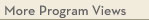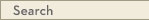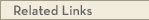# University Catalogs

Twin Cities campusTwin Cities Campus

# Mathematics Minor

CLA Dean's Office
College of Liberal Arts
• Program Type: Undergraduate minor related to major
• Requirements for this program are current for Spring 2015
• Required credits in this minor: 24 to 28
Students complete all the lower-division requirements in the mathematics major, plus two upper-division electives. See the mathematics major description for information on the utility of a mathematics degree.
Program Delivery
This program is available:
• via classroom (the majority of instruction is face-to-face)
Minor Requirements
Students may earn no more than one undergraduate degree in mathematics: a B.A. or a B.S. or a minor.
Minor Courses
Note: qualified honors students can substitute MATH 3592H & 3593H for MATH 2243/2373/2574H, MATH 2263/2374/2573H, and MATH 2283/3283W. See your UHP adviser for final consent. Those who take the MATH 3592H & 3593H series will need to complete a minimum total of 24 credits for the minor. Those who do not complete this honors series will need to complete a minimum total of 25 credits for the minor.
Calculus I
MATH 1271 - Calculus I [MATH] (4.0 cr)
or MATH 1371 - CSE Calculus I [MATH] (4.0 cr)
or MATH 1571H - Honors Calculus I [MATH] (4.0 cr)
Calculus II
MATH 1272 - Calculus II (4.0 cr)
or MATH 1372 - CSE Calculus II (4.0 cr)
or MATH 1572H - Honors Calculus II (4.0 cr)
Linear Algebra & Differential Equations
MATH 2243 - Linear Algebra and Differential Equations (4.0 cr)
or MATH 2373 - CSE Linear Algebra and Differential Equations (4.0 cr)
or MATH 2574H - Honors Calculus IV (4.0 cr)
Multivariable Calculus
MATH 2263 - Multivariable Calculus (4.0 cr)
or MATH 2374 - CSE Multivariable Calculus and Vector Analysis (4.0 cr)
or MATH 2573H - Honors Calculus III (4.0 cr)
Sequences, Series, & Foundations
MATH 2283 - Sequences, Series, and Foundations (3.0 cr)
or MATH 3283W - Sequences, Series, and Foundations: Writing Intensive [WI] (4.0 cr)
Electives
Selection of MATH course electives for the minor requires department adviser approval.
MATH 4xxx-5xxx
Take 2 or more course(s) totaling 6 or more credit(s) from the following:
· MATH 4xxx
· MATH 5xxx
or Theory of Statistics
STAT 5101 - Theory of Statistics I (4.0 cr)
STAT 5102 - Introduction to Statistical Learning (4.0 cr)View college catalog(s): · College of Liberal Arts View future requirement(s): · Spring 2020 · Fall 2018 · Fall 2016 View checkpoint chart: · Mathematics Minor View PDF Version:Search Programs Search University CatalogsOne Stop for tuition, course registration, financial aid, academic calendars, and moreMATH 1271 - Calculus I (MATH)
 Credits: 4.0 [max 4.0] Course Equivalencies: 00067 - Math 1271/Math 1281/Math 1371/ Typically offered: Every Fall, Spring & Summer
Differential calculus of functions of a single variable, including polynomial, rational, exponential, and trig functions. Applications, including optimization and related rates problems. Single variable integral calculus, using anti-derivatives and simple substitution. Applications may include area, volume, work problems. prereq: 4 yrs high school math including trig or satisfactory score on placement test or grade of at least C- in [1151 or 1155]
MATH 1371 - CSE Calculus I (MATH)
 Credits: 4.0 [max 4.0] Course Equivalencies: Math 1142/1271/1281/1371/1571H Typically offered: Every Fall & Spring
Differentiation of single-variable functions, basics of integration of single-variable functions. Applications: max-min, related rates, area, curve-sketching. Use of calculator, cooperative learning. prereq: CSE or pre-bioprod concurrent registration is required (or allowed) in biosys engn (PRE), background in [precalculus, geometry, visualization of functions/graphs], instr consent; familiarity with graphing calculators recommended
MATH 1571H - Honors Calculus I (MATH)
 Credits: 4.0 [max 4.0] Course Equivalencies: 00067 - Math 1142/1271/1281/1371/1571H Grading Basis: A-F only Typically offered: Every Fall
Differential/integral calculus of functions of a single variable. Emphasizes hard problem-solving rather than theory. prereq: Honors student and permission of University Honors Program
MATH 1272 - Calculus II
 Credits: 4.0 [max 4.0] Course Equivalencies: Math 1272/1282/1252/1372/1572 Typically offered: Every Fall, Spring & Summer
Techniques of integration. Calculus involving transcendental functions, polar coordinates. Taylor polynomials, vectors/curves in space, cylindrical/spherical coordinates. prereq: [1271 or equiv] with grade of at least C-
MATH 1372 - CSE Calculus II
 Credits: 4.0 [max 4.0] Course Equivalencies: Math 1272/1282/1252/1372/1572 Typically offered: Every Spring
Techniques of integration. Calculus involving transcendental functions, polar coordinates, Taylor polynomials, vectors/curves in space, cylindrical/spherical coordinates. Use of calculators, cooperative learning. prereq: Grade of at least C- in [1371 or equiv], CSE or pre-Bioprod/Biosys Engr
MATH 1572H - Honors Calculus II
 Credits: 4.0 [max 4.0] Course Equivalencies: 00068 - Math 1272/1282/1252/1372/1572 Grading Basis: A-F only Typically offered: Every Spring
Continuation of 1571. Infinite series, differential calculus of several variables, introduction to linear algebra. prereq: 1571H, honors student, permission of University Honors Program
MATH 2243 - Linear Algebra and Differential Equations
 Credits: 4.0 [max 4.0] Course Equivalencies: 00561 Typically offered: Every Fall, Spring & Summer
Linear algebra: basis, dimension, matrices, eigenvalues/eigenvectors. Differential equations: first-order linear, separable; second-order linear with constant coefficients; linear systems with constant coefficients. prereq: [1272 or 1282 or 1372 or 1572] w/grade of at least C-
MATH 2373 - CSE Linear Algebra and Differential Equations
 Credits: 4.0 [max 4.0] Course Equivalencies: Math 2243/2373/2573 Typically offered: Every Fall & Spring
Linear algebra: basis, dimension, eigenvalues/eigenvectors. Differential equations: linear equations/systems, phase space, forcing/resonance, qualitative/numerical analysis of nonlinear systems, Laplace transforms. Use of computer technology. prereq: [1272 or 1282 or 1372 or 1572] w/grade of at least C-, CSE or pre-Bio Prod/Biosys Engr
MATH 2574H - Honors Calculus IV
 Credits: 4.0 [max 4.0] Course Equivalencies: 00561 - Math 2243/Math 2373/Math 2573H Grading Basis: A-F only Typically offered: Every Spring
Advanced linear algebra, differential equations. Additional topics as time permits. prereq: Math 1572H or Math 2573H, honors student and permission of University Honors Program
MATH 2263 - Multivariable Calculus
 Credits: 4.0 [max 4.0] Course Equivalencies: 00069 Typically offered: Every Fall, Spring & Summer
Derivative as linear map. Differential/integral calculus of functions of several variables, including change of coordinates using Jacobians. Line/surface integrals. Gauss, Green, Stokes Theorems. prereq: [1272 or 1372 or 1572] w/grade of at least C-
MATH 2374 - CSE Multivariable Calculus and Vector Analysis
 Credits: 4.0 [max 4.0] Course Equivalencies: Math 2263/2373/2573H/3251 Typically offered: Every Fall & Spring
Derivative as linear map. Differential/integral calculus of functions of several variables, including change of coordinates using Jacobians. Line/surface integrals. Gauss, Green, Stokes theorems. Use of computer technology. prereq: [1272 or 1282 or 1372 or 1572] w/grade of at least C-, CSE or pre-Bioprod/Biosys Engr
MATH 2573H - Honors Calculus III
 Credits: 4.0 [max 4.0] Course Equivalencies: 00069 - Math 2263/2374/3251 Grading Basis: A-F only Typically offered: Every Fall
Integral calculus of several variables. Vector analysis, including theorems of Gauss, Green, Stokes. prereq: Math 1572H or Math 2574H, honors student and permission of University Honors Program
MATH 2283 - Sequences, Series, and Foundations
 Credits: 3.0 [max 3.0] Course Equivalencies: Math 2283/3283W Typically offered: Every Fall & Spring
Mathematical reasoning. Elements of logic. Mathematical induction. Real number system. General, monotone, recursively defined sequences. Convergence of infinite series/sequences. Taylor's series. Power series with applications to differential equations. Newton's method. prereq: [concurrent registration is required (or allowed) in 2243 or concurrent registration is required (or allowed) in 2263 or concurrent registration is required (or allowed) in 2373 or concurrent registration is required (or allowed) in 2374] w/grade of at least C-
MATH 3283W - Sequences, Series, and Foundations: Writing Intensive (WI)
 Credits: 4.0 [max 4.0] Course Equivalencies: Math 2283/3283W Typically offered: Every Fall & Spring
Introduction to reasoning used in advanced mathematics courses. Logic, mathematical induction, real number system, general/monotone/recursively defined sequences, convergence of infinite series/sequences, Taylor's series, power series with applications to differential equations, Newton's method. Writing-intensive component. prereq: [concurrent registration is required (or allowed) in 2243 or concurrent registration is required (or allowed) in 2263 or concurrent registration is required (or allowed) in 2373 or concurrent registration is required (or allowed) in 2374] w/grade of at least C-
STAT 5101 - Theory of Statistics I
 Credits: 4.0 [max 4.0] Typically offered: Every Fall
Logical development of probability, basic issues in statistics. Probability spaces. Random variables, their distributions and expected values. Law of large numbers, central limit theorem, generating functions, multivariate normal distribution. prereq: [Math 2263 or Math 2374 or Math 2573H], [CSCI 2033 or Math 2373 or Math 2243]
STAT 5102 - Introduction to Statistical Learning
 Credits: 4.0 [max 4.0] Course Equivalencies: 00260 Typically offered: Every Spring
Sampling, sufficiency, estimation, test of hypotheses, size/power. Categorical data. Contingency tables. Linear models. Decision theory. prereq: [5101 or Math 5651 or instr consent]